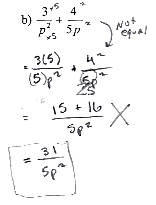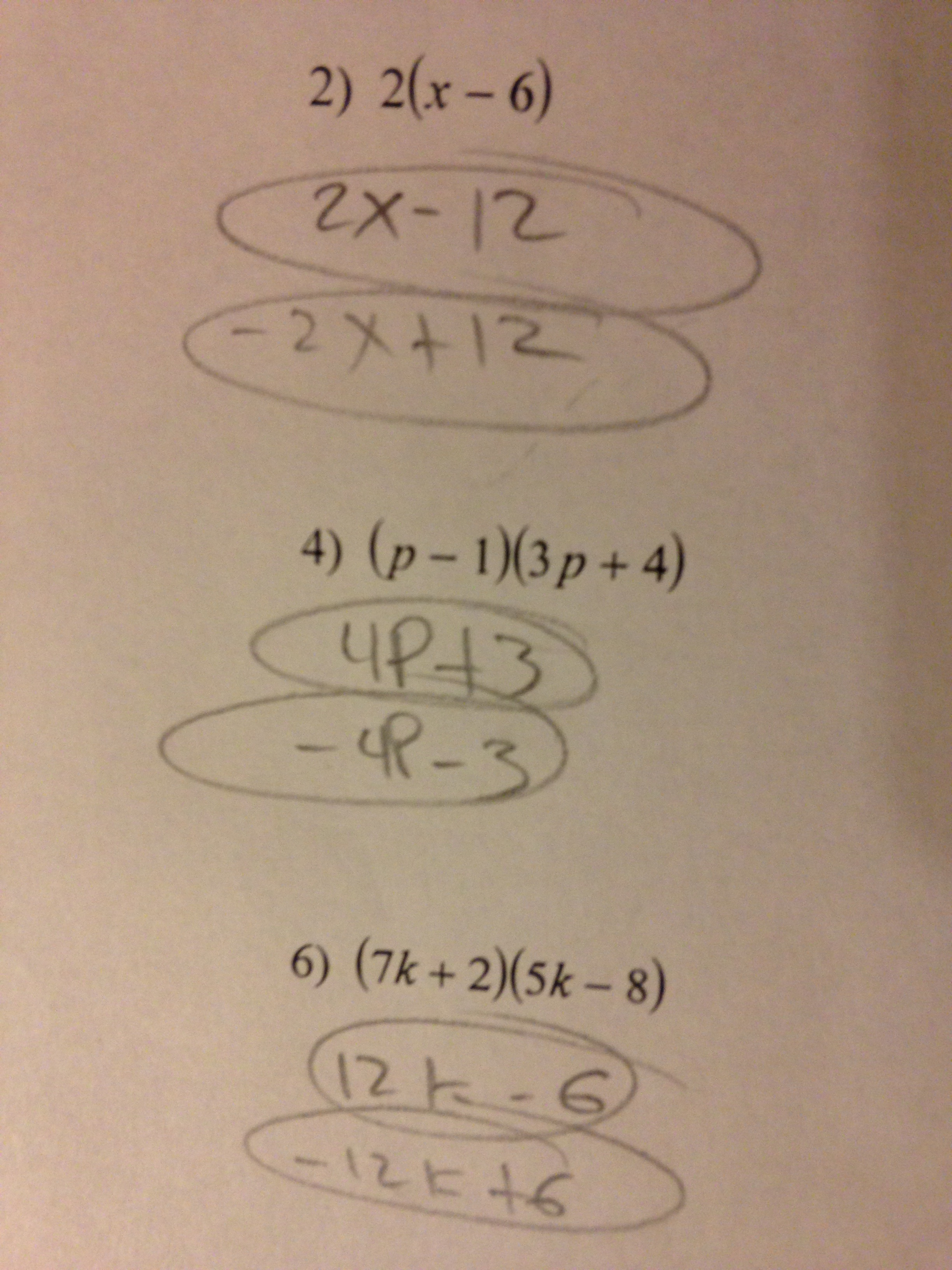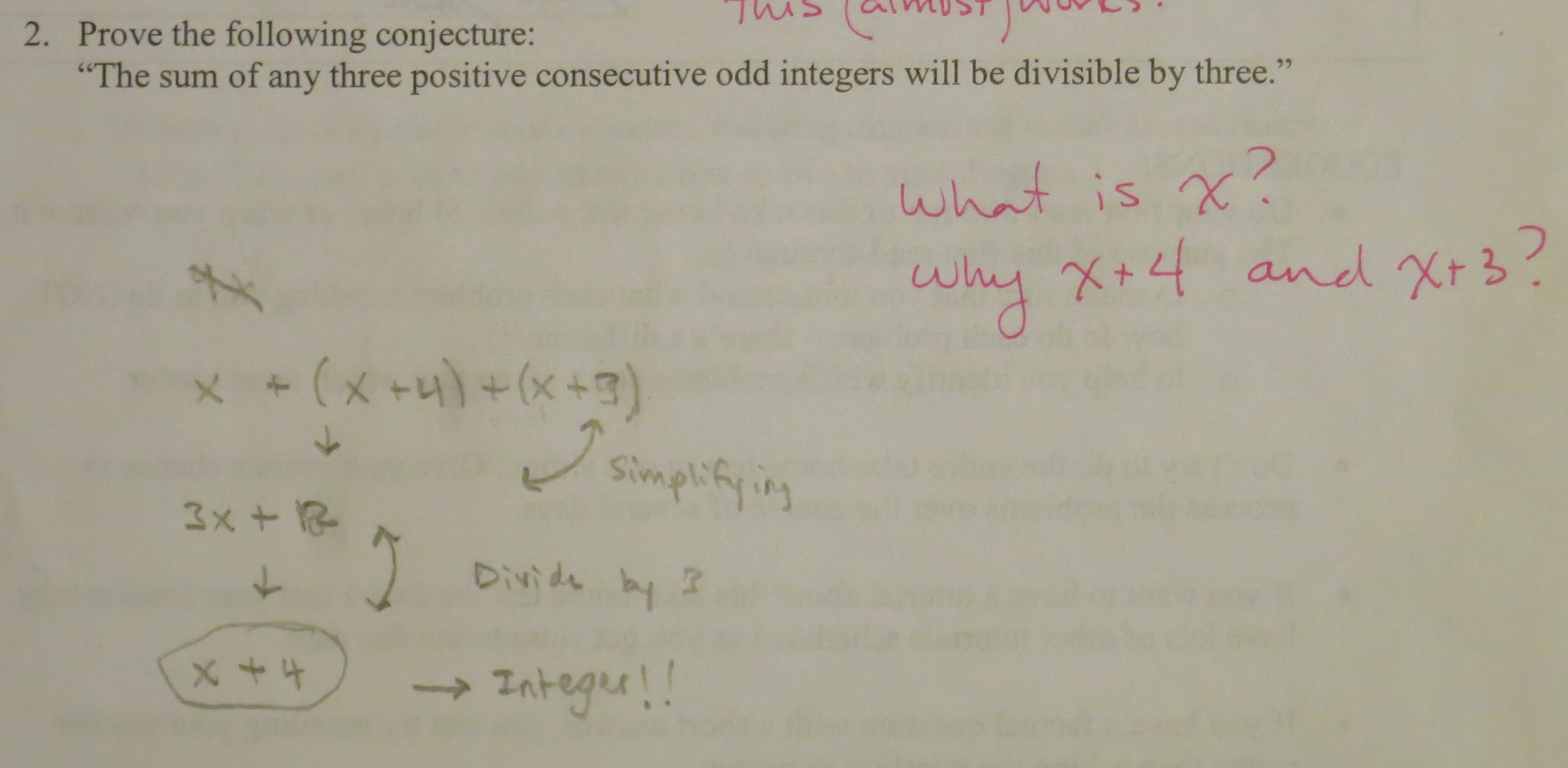Categories

## Constructing Trinomials – Where to go Next?

Hi everyone,

My name is Bryan Penfound. Awhile back I was asked if I would be interested in helping out at MathMistakes and I said yes not knowing how challenging this term would be for me. Now that I have settled in a little bit, I thought I was a bit overdue for a post, so here goes!

Recently while volunteering at a local high school in a grade 9 classroom, I had to opportunity to observe students’ answers to the following question: “Create a trinomial in the variable t that has degree 3 and a constant term of -4.”

Here are five of my favourite responses:I would love to get some discussion going. Choose one of the polynomials above and try to deconstruct what the student knows and what the student still has misconceptions about. What follow-up questions might you ask to learn more information about how the student is thinking? What follow-up questions might you ask to help with any current misconceptions?

Categories

## Squaring doesn’t make equivalent fractionsSquaring doesn’t make equivalent fractions.

Thanks again to Gregory Taylor for the submission.

Categories

## Inconsistent Polynomial MultiplicationWhy does this student answer$2a + 9$ for the first question, and$x + 49$ for the second questions?

Why does this student express more confidence on the first question than the second question?

This post is part of a series analyzing a bunch of survey results. For previous posts, go here, here and here.

Categories

## Diving into the Multiplying Polynomials SurveyThis is from yesterday’s survey, which was discussed over at this post. What do you make of the responses, particularly the differences between  (2a+6) in the first response, and (2x+49) in the second?

This post is part of a series analyzing a bunch of survey results. For previous posts, go here and here.

Categories

## Another pre-instruction survey, this time about polynomial multiplication

View this document on Scribd

Noteworthy:

• The kids have a ton of confidence, even in the stuff that they haven’t formally studied in class yet.  (For this survey, Questions 1-3 had been covered formally, and Questions 4-5 had not.) To my mind, this continues to reaffirm that the most annoying mistakes aren’t the distortion of instruction; they’re the failure of instruction to override preconceptions.
• Kids like to say that$(x+7)^2 = 49$, and teachers like to say that this is due to overuse of the Distributive Property. That might be true, but those teachers also have to recognize that kids said that$(a+3)(a+3)=a^2 + 9$ with almost the same verve and frequency. It’s hard to blame exponents or notation for that mistake, right? So where does this intuition come from?
• A couple of kids included a$2a$ term in Q4 and a$x^2$ term in Q5. I find this interesting, but I’m not exactly sure what its significance is. Is the temptation to add$a+3$ and$a+3$ when the binomials are in the same visual position that they are for addition problems?

The idea that kids walk into our classes with these intuitions is, I think, counter to the way that most math teachers talk and think about these mistakes. I think that realizing that these mistakes are the result of deep intuitions about how math should be is important. I also think thinking about where these intuitions come from is important, because maybe we can avoid setting them in earlier years.

I hope that some of you will give this survey to your students who haven’t yet received instruction on how to multiply polynomials. The original survey can be found here.

You’ll disagree with me in the comments, right? I’m counting on you all…

Categories

## Multiplying monomials and binomialsThere’s a lot going on here. What about this work do you find the most interesting? How would you help this student?

Thanks to Heather for the submission!

Categories

## “Prove the following conjecture: The sum of any 3 positive consecutive odd integers will be divisible by 3.”First, I just love the question. Second: theorize! What happened to this student? What are some good signs in the student’s work?

Thanks to Bree  for the submission.

Also: I categorized this under “Standards for Mathematical Practice.” Does anyone have another CCSS standard that fits this problem and work?

Categories

## PEMDAS and powers

Question: Evaluate the expression$-z^{2} + x(3-y)^2$  when$x = 10$,   \$latex y = -2\$, and  \$latex z = -2\$.

What happened?  First, I HATE PEMDAS AND ANYONE WHO USES IT.  This starts early, and students are already brainwashed by 6th grade when I get them.  All of the GEMS in the world can’t seem to fix this.  I hate PEMDAS because students see parenthesis and go into “I must do that first” mode, even when there is only ONE number inside the parenthesis.  Just because it is in parenthesis, one number, for example (2), does NOT a group make.

Discuss her evaluation of the problem, and her next steps, either in the comments or at her place.

Categories

## Distributive Property, Again!What task or problem would you have this student attempt? Or would you follow this up with an explanation?

Thanks to John Weisenfeld for the submission!

Categories

## Combining like terms……is probably looking like an unfortunate name for adding monomials when you see a mistake like this one. Right?

Or wrong? Speak up in the comments. And more thanks to John Weisenfeld for the mistake.